总结python的常见矩阵运算！(矩阵的创建，numpy，应元素相乘）

74 篇文章 0 订阅

python的numpy库提供矩阵运算的功能，因此我们在需要矩阵运算的时候，需要导入numpy的包。

from numpy import *;#导入numpy的库函数
import numpy as np; #这个方式使用numpy的函数时，需要以np.开头。

from numpy import *;
a1=array([1,2,3]);
a1=mat(a1);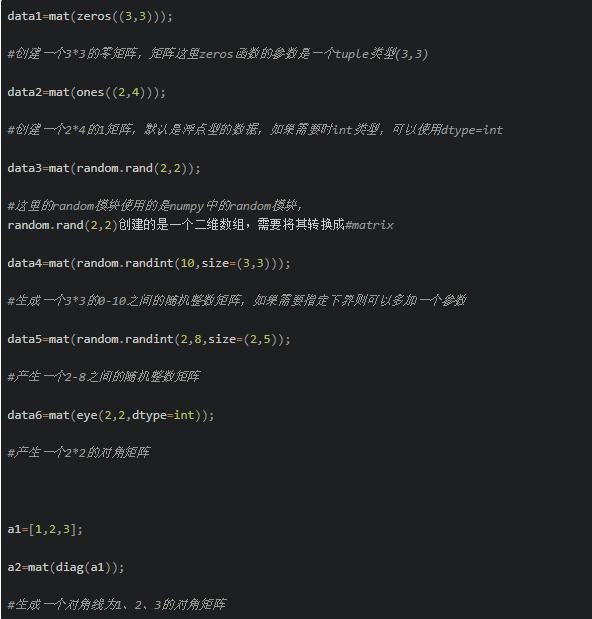1. 矩阵相乘

a1=mat([1,2]);
a2=mat([,]);
a3=a1*a2;
#1*2的矩阵乘以2*1的矩阵，得到1*1的矩阵

2. 矩阵点乘

a1=mat([1,1]);
a2=mat([2,2]);
a3=multiply(a1,a2);

a1=mat([2,2]);
a2=a1*2;

3.矩阵求逆，转置

a1=mat(eye(2,2)*0.5);
a2=a1.I;
#求矩阵matrix([[0.5,0],[0,0.5]])的逆矩阵

a1=mat([[1,1],[0,0]]);
a2=a1.T;

4.计算矩阵对应行列的最大、最小值、和。

a1=mat([[1,1],[2,3],[4,2]]);

a2=a1.sum(axis=0);//列和，这里得到的是1*2的矩阵
a3=a1.sum(axis=1);//行和，这里得到的是3*1的矩阵
a4=sum(a1[1,:]);//计算第一行所有列的和，这里得到的是一个数值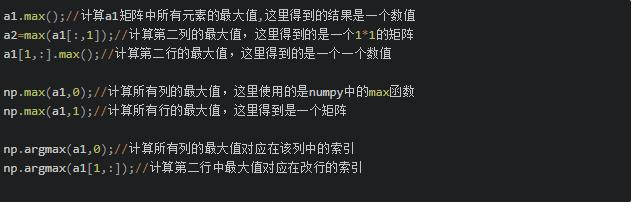5.矩阵的分隔和合并

a=mat(ones((3,3)));
b=a[1:,1:];//分割出第二行以后的行和第二列以后的列的所有元素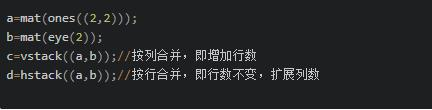l1=[,'hello',3];

numpy中数组，同一个数组中所有元素必须为同一个类型，有几个常见的属性：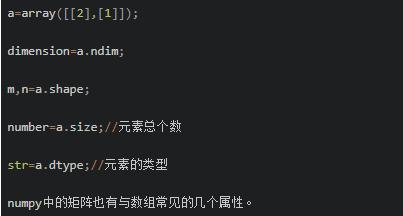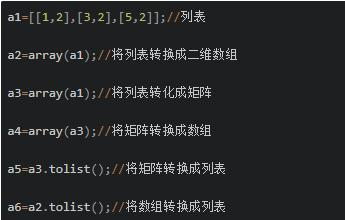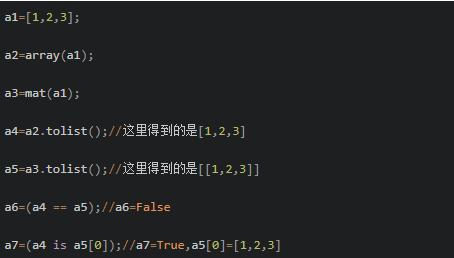dataMat=mat();
val=dataMat[0,0];//这个时候获取的就是矩阵的元素的数值，而不再是矩阵的类型

09-201万+
03-253772

05-142万+
02-204926
12-042922
12-182959
01-032万+
09-132621
09-253万+
12-10156
11-24367
07-123835
03-011197
04-144576
11-091110
06-182481
04-032万+
11-261万+
02-095万+
05-215912¥2 ¥4 ¥6 ¥10 ¥20余额支付 (余额：-- )扫码支付获取中扫码支付点击重新获取扫码支付1.余额是钱包充值的虚拟货币，按照1:1的比例进行支付金额的抵扣。
2.余额无法直接购买下载，可以购买VIP、C币套餐、付费专栏及课程。余额充值